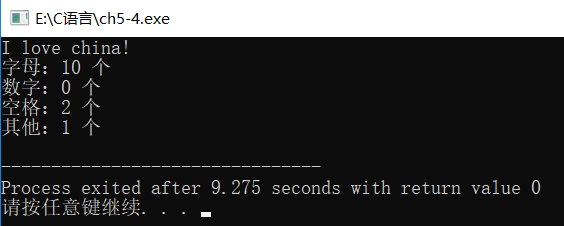## C 语言 | 选择结构程序设计, 第12题

10m，塔以外无建筑物。今输入任何一点的坐标，求该点的建筑高度（塔外的高度为 0m）

# C 语言代码：

``````#include <stdio.h>
#include <math.h>

int main() {
double x,y,d1,d2,d3,d4;

printf("请输入坐标：");
scanf("%lf %lf", &x, &y);

d1 = sqrt(pow(x-2, 2)+pow(y-2, 2));//第一象限    2, 2
d2 = sqrt(pow(x+2, 2)+pow(y-2, 2));//第二象限   -2, 2
d3 = sqrt(pow(x+2, 2)+pow(y+2, 2));//第三象限   -2,-2
d4 = sqrt(pow(x-2, 2)+pow(y+2, 2));//第四象限    2,-2

if(d1<=1 || d2<=1 || d3<=1 || d4<=1) {
printf("该点的建筑高度是 10m");
} else {
printf("该点的建筑高度是 0m");
}

return 0;
}``````

# 运行结果：

``````请输入坐标：1.5 2.3
x=1.500000      y=2.300000

--------------------------------
Process exited after 7.869 seconds with return value 0

## C语言 | 给出一个不多于5位的正整数，求它是几位数，分别输出每一位数字，按逆序输出各位数字

9.给出一个不多于5位的正整数：
① 求出它是几位数；
② 分别输出它是几位数；
③ 按逆序输出个位数字，例如原数为 321，应输出 123。

# 大致思路：

1. 要求判断它是几位数，那么我们可以先求出各个数对应位置的值（个、十、百、千、万），具体取法看下方 `C 语言代码`
2. 开始判断

1. 从一个数（假设是：12345）的 万位 开始判断，如果不是 0，将万位的值赋给 `a 变量`，并 `i=i+1`
2. 然后判断 千位，如果不是 0，将千位的值赋给 `b 变量`，并 `i=i+1`
3. 然后判断 百位，如果不是 0，将百位的值赋给 `c 变量`，并 `i=i+1`
4. 然后判断 十位，如果不是 0，将十位的值赋给 `d 变量`，并 `i=i+1`
5. 然后判断 个位，如果不是 0，将个位的值赋给 `e 变量`，并 `i=i+1`
3. 然后输出变量 `i` 的值，即可得到这个数是几位数
4. 分别输出每一个数字，即先判断它几位数，然后输出 a、b、c、d、e 变量的值即可，具体可以看下方代码
5. 逆序输出这个数字，先判断几位数，再输出 a、b、c、d、e 变量的值，具体可以看下方代码

- 阅读剩余部分 -

# 注意：向上/向下 取整，使用的是 `math.h` 里面的 `ceil` / `floor` 函数，故需要引入头文件 `math.h`

## 1. 向上取整，使用 `ceil` 函数

`ceil()` 向上取整，即求不小于某个数的最小整数，其原型为：
`double ceil (double x);`

【参数】x 为一个指定的数。
【返回值】返回一个不小于 x 的最小整数。

- 阅读剩余部分 -

# C语言代码如下：

``````#include <stdio.h>

int main() {
char c;
int alpha=0, number=0, space=0, other=0;

c = getchar();//输入一个字符给变量 c
while(c != '\n'){
if((c>='A' && c<='Z') || (c>='a' && c<='z')){//判断是否为字母
alpha = alpha+1;
}else if(c>='0' && c<='9'){//判断是否为数字
number = number+1;
}else if(c == ' '){//判断是否为空格
space = space+1;
}else{//其他
other = other+1;
}
c = getchar();//在输入下一个字符赋值给变量 c
}

printf("字母：%d 个\n", alpha);
printf("数字：%d 个\n", number);
printf("空格：%d 个\n", space);
printf("其他：%d 个\n", other);
}``````

# 运行结果

``````I love china!

--------------------------------
Process exited after 9.275 seconds with return value 0``````## C 语言求1000以内的完数

``````#include <stdio.h>
#include <math.h>

int main() {
int i, a, b;

for(i=1; i<=1000; i++) {
b=0;
for(a=1; a<=i/2; a++){
if(i%a == 0){
b=b+a;//把求出的因子都加起来
}
}
if(b == i){
printf("%d its factors are ", i);
for(a=1; a<=i/2; a++){
if(i%a == 0){
printf(",%d", a);
}
}
printf("\n");
//printf("%d 是完数\n", i);
}
}

}``````